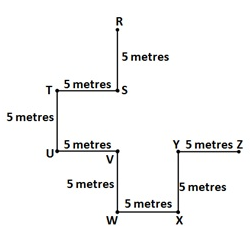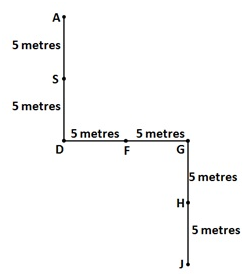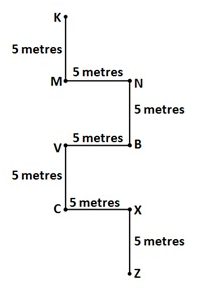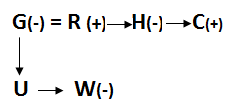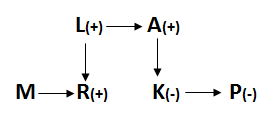# IBPS Clerk Mains Reasoning (Day-04)

Dear Aspirants, Our IBPS Guide team is providing new series of Reasoning Questions for IBPS Clerk Mains 2021 so the aspirants can practice it on a daily basis. These questions are framed by our skilled experts after understanding your needs thoroughly. Aspirants can practice these new series questions daily to familiarize with the exact exam pattern and make your preparation effective.

Start Quiz

Unknown circular seating with distance

Directions (1-5): Study the following information carefully and answer the below questions.

Certain number of persons are sitting in the circular table. The circumference of the table is 360cm. Distance between each person is a consecutive multiple of 3. All of them are facing outside the table. Four persons sit between H and E. Distance between M and L is 39cm. B sits immediate right of M. Three persons sit between B and J. E sits 2nd from J. G is adjacent to J and distance between both is divisible by 5. Two persons sit between H and I. A sits 4th to the right of C and also sits immediate right of I. Distance between C and D is 90cm. Two persons sit between K and O. Distance between O and N is equal to the distance between C and D. More than three persons sit between F and N.

1) How many persons sit between A and D from the side which has the maximum distance between them?

A.Four

B.Two

C.Three

D.Seven

E.None of these

2) How far is B from the left of G?

A.52cm

B.65cm

C.72cm

D.66cm

E.None of these

3) Distance between the left of A to M is same as between with _____?

A.K and B

B.A and I

C.C and D

D.H and N

E.None of these

4) How many persons are sitting in the circular table?

A.18

B.15

C.21

D.20

E.None of these

5) How many perfect square distance persons sitting adjacent to each other are there in the circular table?

A.Three

B.One

C.Four

D.Two

E.None of these

Coded direction sense

Direction (6-8): Read the following information carefully and answer the questions given below.

‘P @ Q’ means ‘P stands 5 metres to the North of Q’

‘P # Q’ means ‘P stands 5 metres to the South of Q’

‘P % Q’ means ‘P stands 5 metres to the East of Q

‘P \$ Q’ means ‘P stands 5 metres to the West of Q’

6) In which direction is Z with respect to R from the given expression ‘[email protected]%[email protected][email protected]\$X#Y\$Z’?

A.Southwest

B.Northwest

C.Southeast

D.Northeast

E.North

7) In which direction is S with respect to J from the given expression ‘[email protected]@[email protected]@J’?

A.Southwest

B.Northwest

C.Southeast

D.Northeast

E.North

8) In which direction is C with respect to M from the given expression ‘Z#X%C#V\$B#N%M#K’?

A.South

B.Northwest

C.Southeast

D.Northeast

E.North

Coded blood relation

Direction (9-10): Read the following information carefully and answer the questions given below.

P @ Q means Q is Mother of P

P # Q means Q is Father of P

P \$ Q means Q is Sister of P

P % Q means Q is Brother of P

P * Q means Q is Son of P

P ! Q means Q is Daughter of P

9) How was G related to H?

Statement: U @ G ! W # R \$ H % C

A.Sister

B.Sister-in-law

C.Brother-in-law

D.Niece

E.None of these

10) How was R related to P?

Statement: M % R # L % A ! K \$ P

A.Sister

B.Brother

C.Cousin

D.Niece

E.None of these

Directions (1-5):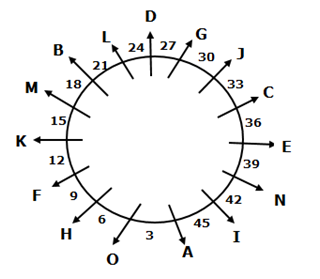Certain number of persons sits in the circular table. The circumference of the table is 360cm. Distance between each person is a consecutive multiple of 3. All of them are facing outside the table.

So the possible of cases is 3,6,9,12,15,18,21,24,27,30,33,36,39,42,45.15 number of persons in the circular arrangement.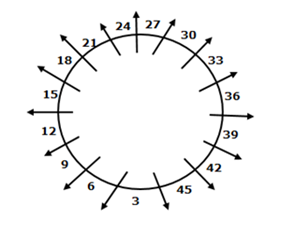Distance between M and L is 39cm. B sits immediate right of M.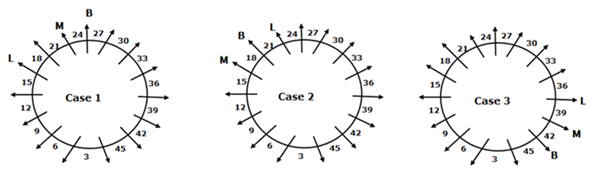Three persons sit between B and J. G is adjacent to J and distance between both is divisible by 5. In thelast statement possibility are 15, 30 or 45.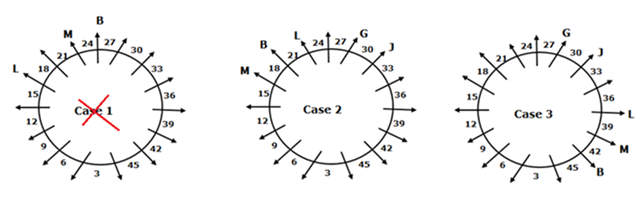E sits 2nd from J. Two persons sit between H and I. Four persons sit between H and E. A sits 4th to the right of C and also sits immediate right of I.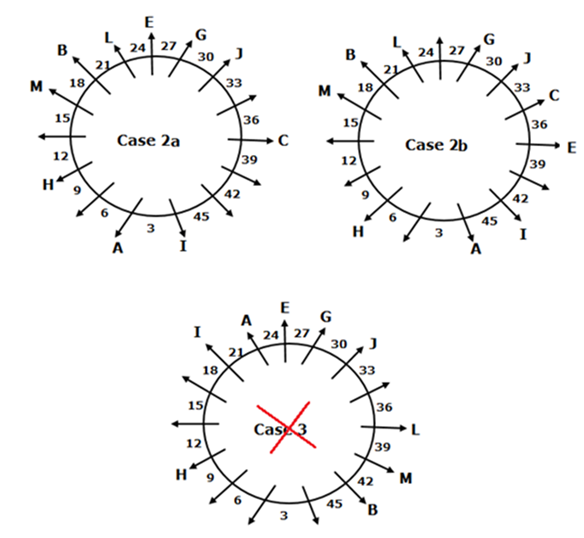Distance between C and D is 90cm. Distance between O and N is equal to the distance between C and D. Two persons sit between K and O. More than three persons sit between F and N.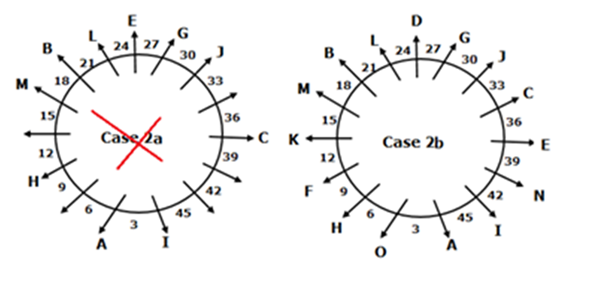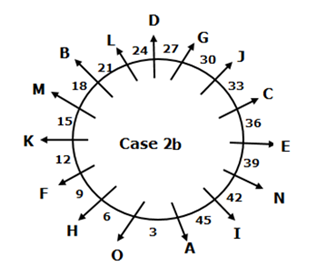Directions (6-8):